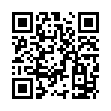# North Coast Synthesis Ltd.

## C♯+2+4+♯2Choose audio sample set:
MSK 007 Leapfrog VCF (patch; see in online store)
MSK 009 Coiler VCF (patch; see in online store)

Equivalent chord symbols: C♯+4+9+♯2, D♭+2+4+♯2, D♭+4+9+♯2, C♯+2+11+♯2, D♭+2+11+♯2.

Notes in this chord: C♯, D♯, D♯♯, E♯, F♯, G♯. Integer notation: {1, 3, 4, 5, 6, 8}.

Nearby chords (one less note): D♭+2+4, D♭+2+♯2, D♭+4+♯2, D♭m+2+4, D♭4+2+♯2, E♭m4+2+♯1.

Nearby chords (one more note): D♭11+♯2, B13-1+♯4, D♭M11+♯2, E♭m11+♯1, D♭+2+4+♯1+♯2, D♭+2+4+♯2+♯4.

Parallel chords (same structure, different root): C+2+4+♯2, D+2+4+♯2, E+2+4+♯2, F+2+4+♯2, G+2+4+♯2, A+2+4+♯2, B+2+4+♯2, C♭+2+4+♯2, D♭+2+4+♯2, E♭+2+4+♯2, F♭+2+4+♯2, G♭+2+4+♯2, A♭+2+4+♯2, B♭+2+4+♯2, D♯+2+4+♯2, E♯+2+4+♯2, F♯+2+4+♯2, G♯+2+4+♯2, A♯+2+4+♯2, B♯+2+4+♯2.

Experimental fretting charts for guitar standard EADGBE tuning (change tuning or instrument):